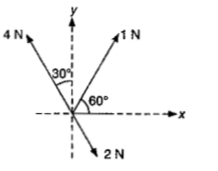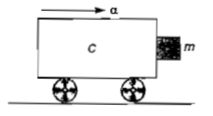The mass of a lift is 2000 kg. When the tension in the supporting cable is 28000 N, its acceleration is

(a)                                       (b)

(c)                                         (d)

Concept Questions :-

Types of forces
High Yielding Test Series + Question Bank - NEET 2020

Difficulty Level:

A body, under the action of a force $\stackrel{\to }{F}=6\stackrel{^}{i}-8\stackrel{^}{j}+10\stackrel{^}{k},$ acquires an acceleration of 1$m{s}^{-2}.$ The mass of this body must be

(a) $20\sqrt{10}kg$                                   (b) 10kg

(c) 20kg                                          (d) $10\sqrt{2}kg$

Concept Questions :-

Newton laws
High Yielding Test Series + Question Bank - NEET 2020

Difficulty Level:

A roller coaster is designed such that riders experience "weightlessness" as they go round the top of a hill whose radius of curvature is 20m. The speed of the car at the top of the hill is between

1. 14m/s and 15m/s

2. 15m/s and 16m/s

3. 16m/s and 17m/s

4. 13m/s and 14m/s

Concept Questions :-

Non-Uniform vertical circular motion
High Yielding Test Series + Question Bank - NEET 2020

Difficulty Level:

Three forces acting on a body are shown in the figure.To have the resultant force only along the y-direction, the magnitude of the minimum additional force needed is(a)0.5N

(b)1.5N

(c)$\frac{\sqrt{3}}{4}$ N

(d) $\sqrt{3}$N

Concept Questions :-

Application of laws
High Yielding Test Series + Question Bank - NEET 2020

Difficulty Level:

A particle of mass m is projected with velocity v making an angle of ${45}^{°}$ with the horizontal. When the particle lands on the level ground the magnitude of the change in its momentum will be

1.  2 mv

2. mv/$\sqrt{2}$

3. mv$\sqrt{2}$

4. zero

Concept Questions :-

Newton laws
High Yielding Test Series + Question Bank - NEET 2020

Difficulty Level:

Sand is being dropped on a conveyor belt at the rate of M kg/s. The force necessary to keep the belt moving with a constant velocity of v m/s will be

1. Mv newton

2. 2 Mv newton

3. $\frac{Mv}{2}$newton

4. zero

Concept Questions :-

Variable mass system
High Yielding Test Series + Question Bank - NEET 2020

Difficulty Level:

A gramophone record is revolving with an angular velocity $\omega .$ A coin is placed at a distance r from the centre of the record. The static coefficient of friction is $\mu .$ The coin will revolve with the record if

1. $r=\mu g{\omega }^{2}$                                       2. $r<\frac{{\omega }^{2}}{\mu g}$

3. $r\le \frac{\mu g}{{\omega }^{2}}$                                         4. $r\ge \frac{\mu g}{{\omega }^{2}}$

Concept Questions :-

Uniform circular motion
High Yielding Test Series + Question Bank - NEET 2020

Difficulty Level:

A block of mass m is in contact with the cart C as shown in the figure.The coefficient of static friction between the block and the cart is $\mu .$The acceleration $\alpha$ of the cart that will prevent the block from falling satisfies

1. $\alpha >\frac{mg}{\mu }$                                       2. $\alpha >\frac{g}{\mu m}$

3. $\alpha \ge \frac{g}{\mu }$                                          4. $\alpha <\frac{g}{\mu }$

Concept Questions :-

Pseudo force
High Yielding Test Series + Question Bank - NEET 2020

Difficulty Level:

A conveyor belt is moving at a constant speed of 2 m/s. A box is gently dropped on it. The coefficient of friction between them is $\mathrm{\mu }=0.5.$ The distance that the box will move relative to the belt before coming to rest on it taking , is

1.                                             2.

3. zero                                               4.

Concept Questions :-

Friction
High Yielding Test Series + Question Bank - NEET 2020

Difficulty Level:

A person of mass 60 kg is inside a lift of mass

940 kg and presses the button on control

panel. The lift starts moving upwards with an

acceleration . If , the tension

in the supporting cable is

1. 9680 N

2. 11000 N

3.  1200 N

4. 8600 N

Concept Questions :-

Types of forces